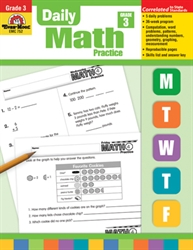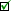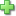# Daily Math Practice Grade 3

Current Retail Price: \$22.99
Used Price: \$8.40 (1 in stock)Condition Policy

See series description for full review.

The skills practiced in Daily Math Practice, Grade 3 include:

Computation

• multiplication & division facts
• multidigit addition & subtraction with regrouping
• multidigit addition & subtraction without regrouping
• multidigit by 1-digit multiplication with regrouping
• multidigit by 1-digit multiplication without regrouping
• 2-digit by 1-digit division with remainders
• 2-digit by 1-digit division without remainders
• word problems

Number

• write number sentences
• write word problems
• read and write number words
• round numbers to nearest thousand
• place value
• count by two, threes, etc.
• odd/even
• ordinal numbers
• estimation
• greater/less than, equal to
• properties, number relationships
• fractions and decimals

Patterns

• create, name, describe, extend

Geometry

• shapes
• symmetry
• congruency
• perimeter
• area
• line segments, angles

Measurement

• weight and capacity
• time
• linear measure
• money

DataClick here to write a review